Next: Magnatohydrostatic Clouds Up: Local Star Formation Process Previous: Equilibria of Cylindrical Cloud   Contents

# Virial Analysis

Hydrodynamic equation of motion using the Lagrangean time derivative [eq.(A.3)] is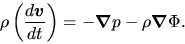(4.17)

Multiplying the position vector r and integrate over a volume of a cloud, we obtain the Virial relation as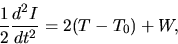(4.18)

where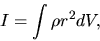(4.19)

is an inertia of the cloud,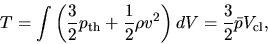(4.20)

is a term corresponding to the thermal pressure plus turbulent pressure,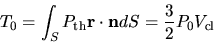(4.21)

comes from a surface pressure, and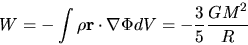(4.22)

is a gravitational energy. To derive the last expression in each equation, we have assumed the cloud is spherical and uniform. Here we use a standard notation as the radius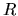, the volume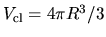, the average pressure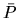, and the mass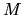.

To obtain a condition of mechanical equilibrium, we assume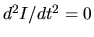. Equation (4.18) becomes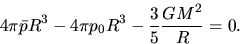(4.23)

Assuming the gas is isothermal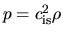, the average pressure is written as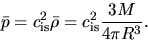(4.24)

Using equation (4.24) to eliminate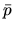from equation (4.18), the external pressure is related to the mass and the radius as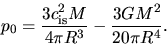(4.25)

Keepingconstant and increasingfrom zero,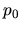increases first, but it takes a maximum,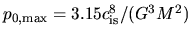, and finally declines. This indicates that the surface pressure must be smaller than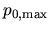for the cloud to be in an equilibrium. In other words, keepingand changing, it is shown thathas a maximum value to have a solution. The maximum mass is equal to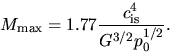(4.26)

The cloud massive than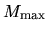cannot be supported against the self-gravity. This corresponds to the Bonnor-Ebert mass [eq.(4.9)], although the numerical factors are slightly different.

SubsectionsNext: Magnatohydrostatic Clouds Up: Local Star Formation Process Previous: Equilibria of Cylindrical Cloud   Contents
Kohji Tomisaka 2007-07-08